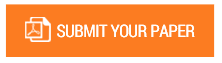New Methods to Solve some Types of the First Order D.E.s

Volume/Issue : Volume 7 - 2022, Issue 3 - March

Scribd : https://bit.ly/3v0wboJ

DOI : https://doi.org/10.5281/zenodo.6476710

There are six Rules to solve the non exact differential equations (D.E.s) so we must learn and keep them and choosethe proper Rule to find theIntegrating Factor [ µ(x,y)] of the D.E. . so we must multiply I.F. by the equation to make it an exactand then integrate it , but sometimes this takes long time , more paper and more mistakes .There are two types of the first order differential equations either an exact or non exact and the exact D.E. can solved ( integrated )directly but for non exact we must find the integrating factor µ(x,y). The exact D.E. usually is the dirivative of a function of one or many functions each one of them is linear and iindependent on the others. But if one function or more are rational or logarthmic so the D.E. becomes non exact. For me I discovred new methods to find the integrating factor in one prcedure to solve many types of the differential equations of the first orderin short time with simple ways (may be without integration ) and this will increase the FLEXIBILITY tochoose the simple and short procedure. There are two types of the non exact differential equations can not solved by my method : The homogeneous D.E. which contents the same term in M & N togerther . The editing D.E. which we can not find the right integrating factor of it from M and N .

Keywords : By checking most of the D.E.s so you may write at least one of its functions directly without integration then from that function you can calculate the integrating

#### CALL FOR PAPERS

Paper Submission Last Date
31 - December - 2023